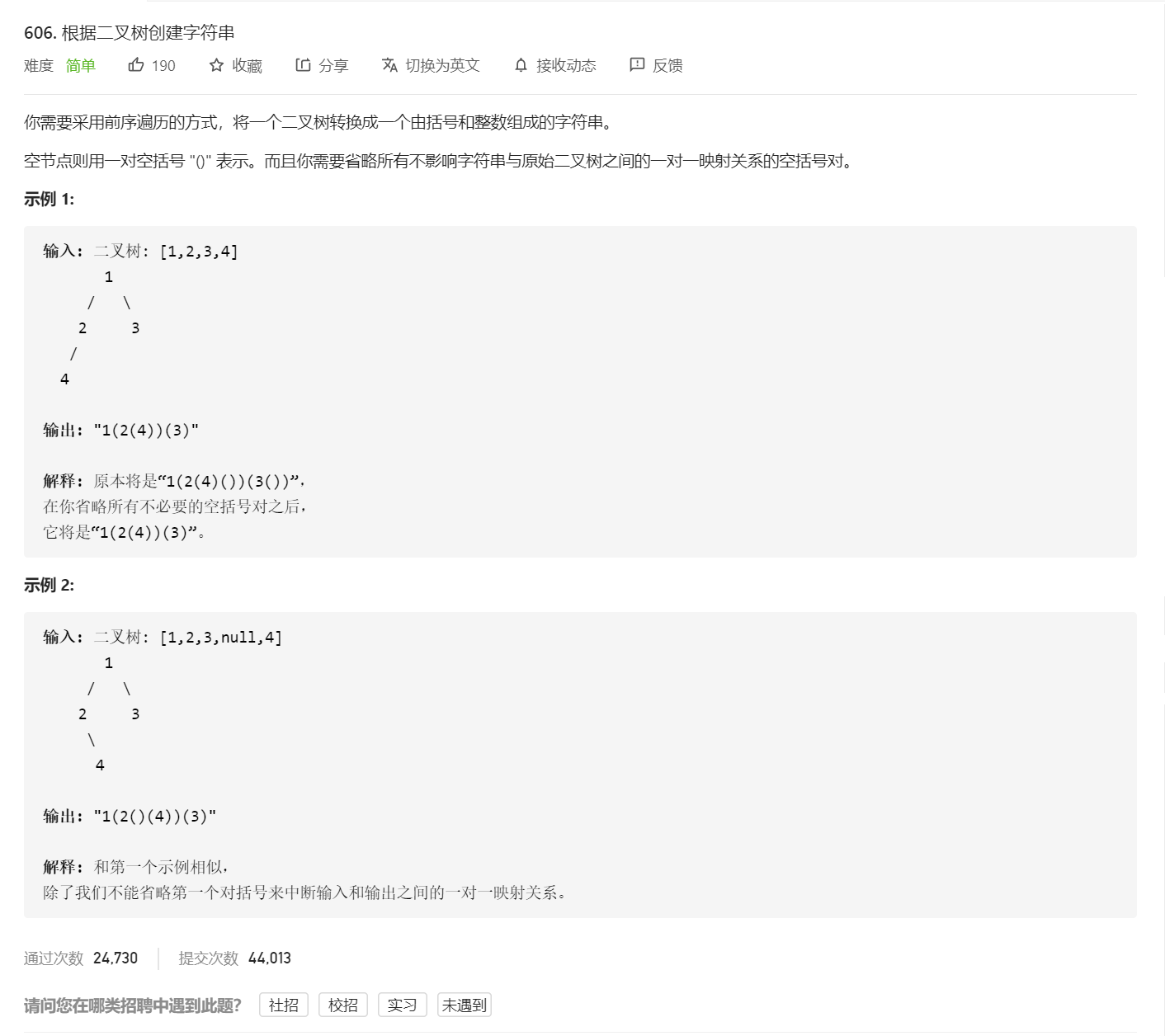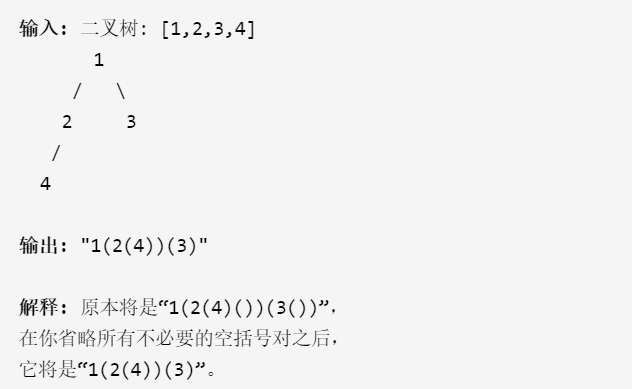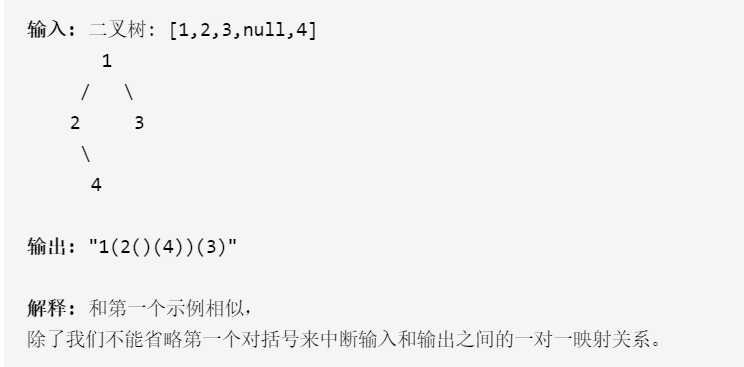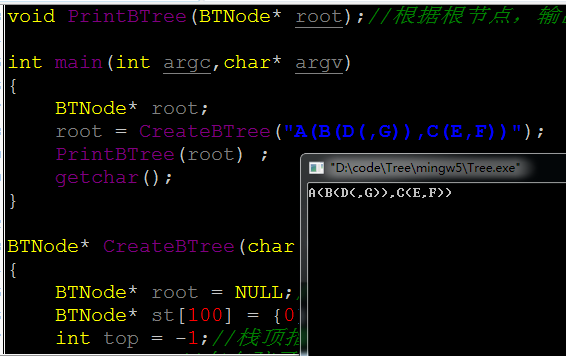• 这道题其实考察到的是二叉树括号表示法括号表示法依靠括号的划分来区分左子树和右子树。整体思路不难，也即使递归创建：首先字符串中加入结点的值，然后遍历左子树和右子树，在遍历每棵子树的时候首先创建(，...
前言：
二叉树刷题是有固定思维的，请移步

README】二叉树刷题框架

根据二叉树创建字符串
题目
点击跳转：LeetCode递归解法
这道题其实考察到的是二叉树的括号表示法，括号表示法依靠括号的划分来区分左子树和右子树。整体思路不难，也即使递归创建：首先字符串中加入结点的值，然后遍历左子树和右子树，在遍历每棵子树的时候首先创建(，然后递归，接着递归完成之后再创建)，对于空结点则表示为（）
也就是如果不要去管题目的其它限制，可以写出这种情况下递归代码
class Solution {
public:
string tree2str(TreeNode* root)
{
string ret;
if(root=nullptr)
return ret;

ret+=to_string(root-val);

ret+='(';//遍历子树先加左括号
ret+=tree2str(root->left);//递归
ret+=')';//遍历完成之后再加右括号

ret+='(';
ret+=tree2str(root->right);
ret+=')';

}
};

这样创建出的字符串是不满足题意的，题目要求的是对于可以省略的括号应该省略。比如下图中结点3由于没有左右子树，所以它的空结点就不创建括号，还有结点2它只有左子树没有右子树，但是右子树写不写括号不影响对应，因此可以不写它右子树的括号但是有些括号不能省略，比如下图中，结点2没有左子树但是拥有右子树，如果此时省略了结点2的左子树的括号，那么在括号表示法中就无法区分4到底是左子树还是右子树了所以综上我们可以写出如下代码
class Solution {
public:
string tree2str(TreeNode* root)
{
string ret;
if(root==nullptr)
{
return ret;
}
ret+=to_string(root->val);
if(root->left==nullptr && root->right==nullptr)
{
return ret;
}

ret+='(';
ret+=tree2str(root->left);
ret+=')';

if(root->right!=nullptr)
{
ret+='(';
ret+=tree2str(root->right);
ret+=')';
}

return ret;

}
};

如果不满足 if(root->left==nullptr && root->right==nullptr)，指的就是就是此时 root->left==nullptr 但是root->right!=nulltptr，这种情况下正好就符合我们的逻辑，左子树递归进去创建括号，右子树进入if判断也创建括号，以此就正确区分了左右子树优化
从刚才代码的提交结构中大家可以看到，时间以及空间效率都及其低下，其实这也是递归的通病，递归的思路很简单。我们在上面的代码中使用的是string，string用的不好会导致大量的深拷贝，因此会严重影响效率。
解决方法就是新建一个没有返回值的递归函数_tree2str，在主函数中建立一个string，通过引用传参即可
class Solution {
public:
void _tree2str(TreeNode* root,string& ret)
{
if(root==nullptr)
return;
ret+=to_string(root->val);

if(root->left==nullptr && root->right==nullptr)
return;
ret+='(';
_tree2str(root->left,ret);
ret+=')';

if(root->right!=nullptr)
{
ret+='(';
_tree2str(root->right,ret);
ret+=')';
}
}

string tree2str(TreeNode* root)
{
string ret;
_tree2str(root,ret);
return ret;

}
};

可以发现，这样写代码的效率得到了很大的提高展开全文数据结构
• 通过字符串（括号表示法创建一个二叉树（C语言实现） 实现步骤 假设采用括号表示法表示的二叉树字符串str是正确的，用ch 扫描str，其中只有四类字符，其处理如下。 若ch=’(’， 源代码 头文件 #include"btree....
通过字符串（括号表示法）创建一个二叉树（C语言实现）

写在前面：该篇文章参考文献：数据结构教程（第五版）  李春葆 主编

实现步骤
假设采用括号表示法表示的二叉树字符串str是正确的，用ch 扫描str，其中只有四类字符，其处理如下。

单个字符：结点的值
（：表示一棵子树的开始
）：表示一棵子树的结束
，：表示一棵右子树的开始

算法设计

先构造根结点N，再构造左子树L,最后构造右子树R
构造右子树R时，找不到N了，所以需要保存N
而括号（子树）是按照最近原则匹配的，所以使用一个栈保存N

ch扫描采用括号表示法表示二叉树的字符串：
A(B(D(,G)),C(E,F))

若ch=’(’：表示前面刚创建的结点p存在孩子结点，需要将其进栈作为栈顶结点，并置空k=1，表示开始处理左孩子结点；
若ch=’)’：表示栈顶结点的左右孩子结点处理完毕，退栈；
若ch=’，’：表示开始处理右孩子结点，置k=2；
其他情况（结点值）：
创建p结点用于存放ch
当k=1时，将p作为栈顶元素的左孩子结点；
当k=2时，将p作为栈顶元素的右孩子结点；

源代码

头文件   btree.h

#pragma once
typedef char ElemType;

typedef struct node {
ElemType data;		//数据元素
struct node *lchild;	//指向左孩子的结点
struct node *rchild;	//指向右孩子的结点
}BTNode;


函数实现  btree.cpp

#include<iostream>
#define MaxSize 20   //最大结点数
#include"btree.h"

void CreateBTree(BTNode *&b, char *str)
{
BTNode *St[MaxSize], *p;   //St数组作为顺序栈，保存双亲结点
int top = -1, k, i= 0;		//top为栈顶指针
char ch;
b = NULL;		//初始时二叉链为空
ch = str[i];
while (ch != '\0')		//循环处理，直到str中的每个字符扫描完毕
{
switch (ch)
{
case '(':top++; St[top] = p; k = 1; break;		//开始处理左孩子结点
case ')':top--;  break;							//栈顶结点的子树处理完毕
case ',': k = 2; break;							//开始处理右孩子结点
default:
p = (BTNode *)malloc(sizeof(BTNode));		//创建一个结点由p指向它
p->data = ch;								//存放结点值
p->lchild = p->rchild = NULL;				//左右指针都设置为空
if (b == NULL)					//尚未设置根结点
b = p;						//p作为根结点
else
{
switch (k)
{
case 1:St[top]->lchild = p; break;		//新建结点作为栈顶结点的左孩子
case 2:St[top]->rchild = p; break;		//新建结点作为栈顶结点的右孩子
}
}
}
i++;
ch = str[i];
}
}

补充：关于二叉树的其他基本算法代码
/*销毁二叉树:释放二叉树b的所有结点分配的空间*/
void DestoryBTree(BTNode *&b)
{
if (b != NULL)
{
DestoryBTree(b->lchild);
DestoryBTree(b->rchild);
free(b);
}
}

/*查找结点：在二叉树b中查找值为x的结点，找到后返回其地址，否则返回NULL*/
BTNode *FindNode(BTNode *b, ElemType x)
{
BTNode *p;
if (b == NULL)
return NULL;
else if (b->data == x)
return b;
else
{
p = FindNode(b->lchild, x);
if (p != NULL)
return p;
else
return FindNode(b->rchild, x);
}
}

/*找孩子结点：直接返回结点p的左孩子或者右孩子地址*/
BTNode *LchildNode(BTNode *p)		//返回结点p的左孩子指针
{
return p->lchild;
}

BTNode *RchildNode(BTNode *p)		//返回结点p的右孩子指针
{
return p->rchild;
}

/*求二叉树的高度：返回二叉树的高度*/
int BTHeight(BTNode *b)
{
int lchildh, rchildh;
if (b == NULL) return 0;		//空树高度为0
else
{
lchildh = BTHeight(b->lchild);		//求左子树的高度
rchildh = BTHeight(b->rchild);		//求右子树的高度
return (lchildh > rchildh) ? (lchildh + 1) : (rchildh + 1);
}
}

/*输出二叉树：输出创建二叉树时输入的字符串*/
void DispBTree(BTNode *b)
{
if (b != NULL)
{
printf("%c", b->data);
if (b->lchild != NULL || b->rchild != NULL)
{
printf("(");
DispBTree(b->lchild);
if (b->rchild != NULL)
printf(",");
DispBTree(b->rchild);
printf(")");
}
}
}




展开全文数据结构 字符串
• //栈表示的节点是怎么连接到根节点的？ } if(k==2){ st[top].rchild=p; } } } j++; ch=arr[j]; } } public void dispbtree(Node1 b){//输出二叉树...java
•  1、根据括号表示法创建链式二叉树  2、根据链式二叉树输出对应的括号表示法 二、效果   三、源代码 #include &lt;stdio.h&gt; #include &lt;stdlib.h&gt;//malloc()函数所在库 typedef ...
数据结构与算法 二叉树链式存储与括号表示法的相互转换

一、简述

1、根据括号表示法创建链式二叉树

2、根据链式二叉树输出对应的括号表示法

二、效果三、源代码

#include <stdio.h>
#include <stdlib.h>//malloc()函数所在库

typedef struct NODE  //树节点
{
char data;//节点
struct NODE* lchild;//指向左孩子
struct NODE* rchild;//指向右孩子
}BTNode;

//函数声明
BTNode* CreateBTree(char *str);//根据括号式创建链式二叉树
void PrintBTree(BTNode* root);//根据根节点，输出二叉树的括号表达式

int main(int argc,char* argv)
{
BTNode* root;
root = CreateBTree("A(B(D(,G)),C(E,F))");
PrintBTree(root) ;
getchar();
}

BTNode* CreateBTree(char *str)//根据括号式创建链式二叉树
{
BTNode* root = NULL;//初始化树，tree根节点
BTNode* st = {0} ,*p;//栈st存放要操作的节点，p指向新节点
int top = -1;//栈顶指示
int k = 0;//左右孩子标志
int j = 0;// str索引

char ch =  str[j];//取str第一个字符
while(ch!='\0')//当ch不为 '\n'时，进行操作
{
switch(ch)
{
case '(':st[++top] = p;//遇到字符'（'，将新节点进栈，合法的字符串里面，'('前面肯定会有节点字符
k = 1;//标志下一个节点处理为当前节点的左孩子
break;
case ')':--top;//遇到字符')'表示当前节点处理完毕，将其出栈
break;
case ',':k = 2;//标志下一个节点处理为当前节点的右孩子
break;
default: p = (BTNode*)malloc(sizeof(BTNode));//节点字符，申请新节点
p->data = ch;//将数据域设置为当前字符
p->lchild = NULL;//指针置空
p->rchild= NULL;//指针置空

if(root == NULL)//如果跟节点为空，将新节点作为根节点
{
root = p;
}
else
{
switch(k)//根据k值将新节点设置为当前操作节点的左孩子或右孩子
{
case 1:st[top]->lchild = p;break;
case 2:st[top]->rchild = p;break;
}
}
break;
}
ch = str[++j] ;//继续处理下一字符
}
return root;
}

void PrintBTree(BTNode* root)//根据根节点，输出二叉树的括号表达式
{
if(root!=NULL)
{
printf("%c",root->data);//打印当前节点
if(root->lchild!=NULL || root->rchild!=NULL)//如果当前节点有孩子
{
printf("(") ;//有孩子必定有左括号
if(root->lchild!=NULL) //如果左孩子不为空，打印左孩子
{
PrintBTree(root->lchild);
}
if(root->rchild!=NULL)//如果右孩子不为空，打印右孩子
{
printf(",");//有右孩子必定有','
PrintBTree(root->rchild);
}
printf(")") ;有孩子必定有右括号
}
}
}



展开全文• #include<iostream> #include<stdlib.h> using namespace std; struct TreeNode {//树节点 char data;//数据域 struct TreeNode *Lchild;//左孩子结点 ...root, char *str) {//创建二叉链 Tr数据结构
• 已知一颗二叉树的中序序列为cbedahgijf，后序序列为cedbhjigfa，给出二叉树的树形表示（用括号法）。 输入 输出 输出带有括号与字母的用括号法表示二叉树。 提示 首先根据中序序列和后序序
• C,(E,F))扫描采用括号表示法表示二叉树的字符串 读到的符号为ch使用一个栈保存双亲结点引入一个变量k 作用是指定其后处理的是左孩子还是右孩子k=1 处理左孩子 k=2 处理右孩子情况如下遇到一个实实在在的字母时创建一...
• 采用括号表示法的字符串创建二叉链表表示的二叉树，实现二叉树的基本运算，如下: (1)用括号表示法输出二叉树； (2)输出节点的左、右孩子节点值； (3)输出二叉树的深度； (4)输出二叉树的宽度； (5)输出二叉树的节点...算法 数据结构 字符串
• 括号表示法字符串构造二叉链： 遍历所给的字符串，当一个元素后面紧跟着一个左括号"("，便代表着它有孩子结点，将这个元素入栈，去构造它的左子树和右子树，遇到","，表示右子树的开始，遇到")"，表示一棵子树的结束...c++
• 1.创建二叉树：根据二叉树括号表示法的字符串生成二叉链存储结构 2.销毁二叉树：释放所有结点分配的空间 3.查找结点 4.查找孩子结点 5.求二叉树高度 6.输出二叉树：用括号表示法 创建二叉树 typedef struct node {...
• //本文档主要实现通过正确的括号表示法表示的二叉树字符串创建二叉树 #include using namespace std; #define LEN 1000 //定义二叉树节点的数据结构 struct BTNode {  char data;  BTNode*left;  ...
• 二叉树的基本算法及遍历（递归）创建二叉树（前序遍历）A(B(D,E(H(J,K(L,M(,N))))),C(F,G(,I)))递归销毁二叉树查找值为x的结点（前序遍历）求某一结点的左右孩子求二叉树的高度以括号表示法输出二叉树（前序遍历）...数据结构与算法
• 问题 h: 二叉树建树题目描述括号表示法表示的二叉树字符串 A(B(D,F(E,)),C(G(,H),I))，建立这棵树，并用先序遍历输出结果。输入无输入。直接定义 char *str="A(B(D,F(E,)),C(G(,H),I))"输出输出 先序遍历...
• 网络上有关二叉树建立的代码比较多，但粗略试了下，多数运行还是有问题，故本文给出了基于嵌套括号表示法二叉树创建代码，如下图二叉树，其括号表示法为 A(B(E,F(J,K),G),C,D(H,I)) 当下面程序运行是 (g++ ...遍历
• 先序创建二叉树 顺序创建二叉树 括号表示法创建二叉树 二叉树的删除 二叉树的括号表示输出 二叉树的遍历
• 创建二叉树CreateBTNode(*b, *str)：根据二叉树括号表示法字符串str生成对应的二叉链存储结构b 销毁二叉链存储结构DestroyBT(*b)：销毁二叉链b并释放空间 查找节点FindNode(*b,x)：在二叉树b中寻找data域值为x的节点...数据结构 指针 c++
• 1、括号表示法创建二叉树 2、实现二叉树的递归遍历算法和非递归遍历算法(非递归算法选做)，依次输出二叉树的先序、中序和后序遍历序列 3、二叉树的遍历遍历二叉树，输出结点值大于C的结点，而小于等于C的结点用*...数据结构
• 说明：需事先利用括号扫描法创建一个二叉链bt，该二叉树bt的括号表示法对应的字符串为"A(B(D(G),H),C(E(F,I)))" #include "stdio.h" #include "stdlib.h" #include "ctype.h" #define Maxsize 20 typedef struct ...数据结构 c语言
• 1：由二叉树括号表示法构造链式二叉树 //由二叉树的括号表示， 创建二叉树，并返回根节点的指针 void CreateBTree( BTNode *&root, char str[])//参数：传入根节点的指针，二叉树的括号表示 { //flag标记其后...存储 null
• 括号表示法表示二叉树字符串，创建二叉树。 */ BSTNode* CreateBSTNode(char *s) { char ch; BSTNode *p=NULL, *b=NULL, *ps[MaxSize]; int top=-1, tag=-1; ch=*s; ...
• java二叉树实现 （简单实现，入门用） /**创建二叉树*/ public BinaryTree createTree(String treeStr); /**寻找结点*/ ... /**输出树（括号表示法）*/ public void disPlayTree(BinaryTree tree);